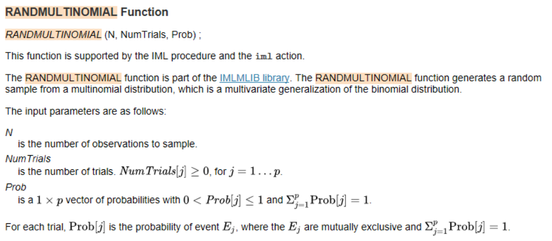## SAS/IML Multinomial distribution with null probabilities in the vector specifying probabilities

Hello,

I'm carrying out a simulation.

I was wondering why this works (I took it from https://blogs.sas.com/content/iml/2013/08/05/simulate-from-multinomial-distribution.html😞

```proc iml;
call randseed(4321);                   /* set random number seed */
c = {"black", "brown", "white"};
prob = {0.5     0.2       0.3};        /* probabilities of pulling each color */
X = RandMultinomial(1000, 100, prob);  /* 1000 draws of 100 socks with 3 colors */
print X[colname=c];```

while this does not:

PROC IML;
call randseed(4321);
c = {"1", "2", "3","4", "5", "6","7", "8", "9","10", "11", "12","13","14"};
prob = {0 0 0.125 0 0.125 0.25 0 0 0.125 0.125 0 0.125 0 0.125 };
X=RandMultinomial(100, 1000, prob);
print X[COLNAME=c];
QUIT;

The log says "ERROR: Matrix X has not been set to a value.".

I assume RandMultinomial can not simulate properly if one of the probabilities is 0. Nevertheless, I can't remove each 0 from the vector, that would be too time-consuming and inconsistent with my goal, I think.

Is there a way to carry out the simulation in SAS/IML keeping the 0's in the vector?

I know I could use the data step-approach reported here

https://blogs.sas.com/content/iml/2016/03/16/simulate-multinomial-sas-data-step.html

but I would like to keep it as simple as possible.

Thanks,

Simone

1 ACCEPTED SOLUTION

Accepted Solutions

## Re: SAS/IML Multinomial distribution with null probabilities in the vector specifying probabilities

You can write a short module that strips out the events that have zero probability. You can return Count=0 for the number of times that those categories occurred:

``````
PROC IML;
/* Return count=0 for categories that have prob=0. */
start RandMult(N, NumTrials, prob);
ncols = prod(dimension(prob));
X = j(N, ncols, 0);
idx = loc(prob>0);     /* only use categories for which prob > 0 */
posProb = prob[idx];
X[ ,idx] = RandMultinomial(N, NumTrials, posProb);
return X;
finish;

call randseed(4321);
c = "1":"14";
prob = {0 0 0.125 0 0.125 0.25 0 0 0.125 0.125 0 0.125 0 0.125 };
X=RandMult(20, 1000, prob);
print X[COLNAME=c];
QUIT;``````

3 REPLIES 3

## Re: SAS/IML Multinomial distribution with null probabilities in the vector specifying probabilities

Hello,

I can only say that the zero-probabilities are not allowed indeed.

From the doc:Thanks,

Koen

## Re: SAS/IML Multinomial distribution with null probabilities in the vector specifying probabilities

You can write a short module that strips out the events that have zero probability. You can return Count=0 for the number of times that those categories occurred:

``````
PROC IML;
/* Return count=0 for categories that have prob=0. */
start RandMult(N, NumTrials, prob);
ncols = prod(dimension(prob));
X = j(N, ncols, 0);
idx = loc(prob>0);     /* only use categories for which prob > 0 */
posProb = prob[idx];
X[ ,idx] = RandMultinomial(N, NumTrials, posProb);
return X;
finish;

call randseed(4321);
c = "1":"14";
prob = {0 0 0.125 0 0.125 0.25 0 0 0.125 0.125 0 0.125 0 0.125 };
X=RandMult(20, 1000, prob);
print X[COLNAME=c];
QUIT;``````

Dear Rick,

Yours sincerely,

Simone

From The DO Loop